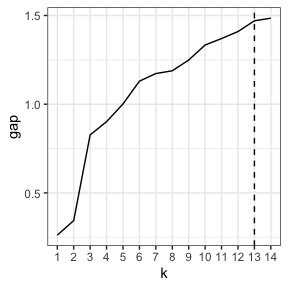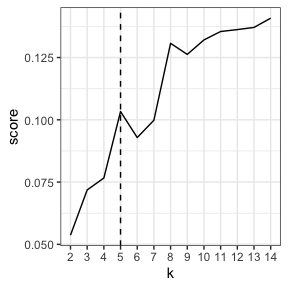# Documenting evaluation

The other vignette focuses on reproducing a single clustering workflow that assumes that the number of clusters has been decided. As the app includes a few options for evaluating clusters, some of the functions are also made available in the package. The output of the clustering functions can also be used with other packages.

library(visxhclust)
library(dplyr)

### Preprocessing and clustering

numeric_data <- iris %>% select(Sepal.Length, Sepal.Width, Petal.Width)
dmat <- compute_dmat(numeric_data, "euclidean", TRUE)
clusters <- compute_clusters(dmat, "complete")

### Gap statistic

For Gap statistic, the optimal number of clusters depends on the method use to compare cluster solutions. The package cluster includes the function cluster::maxSE() to help with that.

gap_results <- compute_gapstat(scale(numeric_data), clusters)
optimal_k <- cluster::maxSE(gap_results$gap, gap_results$SE.sim)
line_plot(gap_results, "k", "gap", xintercept = optimal_k)### Other measures

The Shiny app also includes the option to compute average silhouette widths or Dunn index. The function compute_metric works similarly to compute_gapstat, whereas optimal_score is similar to maxSE. However, optimal_score varies only between first and global minimum and maximum.

res <- compute_metric(dmat, clusters, "dunn")
optimal_k <- optimal_score(res\$score)
line_plot(res, "k", "score", optimal_k)